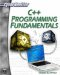# Pointers to Functions

C++ also allows another type of pointer; this one is more useful than pointers to pointers. C++ allows you to create pointers to functions. The greater utility of this is for passing a function as a parameter to another function. That means you can pass an entire function as an argument for another function. To declare a pointer to a function we must declare it like the prototype of the function, but enclosing between parenthesis () the name of the function and then placing a pointer asterisk (*) before the name of the function.

`// pointer to functions #include <iostream> using namespace std;   // function prototypes int subtraction (int a, int b); int addition (int a, int b); //function pointer prototypes int (*minus)(int,int) = subtraction; int operation (int x, int y, int (*functocall)(int,int)) { int i;  i = (*functocall)(x,y);  return (i); } int main () {  int m,n;  m = operation (5, 5, addition);  n = operation (50, m, minus);  cout <<n;  return 0; } int addition (int a, int b) {        int answer;       answer = a + b;       return answer; } int subtraction (int a, int b) {         int answer;      answer = a - b;      return answer; }`

In the example, the word minus is used as a pointer to a function that has two parameters of type int. You can now pass that function’s pointer to another function.

`int (* minus)(int,int) = subtraction;`

In this case, you pass the functions pointer to a function called operation, which will do whatever function is passed to it (be it addition or subtraction). This is a rather powerful feature that C++ provides you.C++ Programming Fundamentals (Cyberrookies)
ISBN: 1584502371
EAN: 2147483647
Year: 2005
Pages: 197
Authors: Chuck Easttom